## Monday, 7 March 2022

### Lupine Publishers| On some Derivatives of Vector-Matrix Products Useful for Statistics

Lupine Publishers| Journal of Biostatistics & Biometrics

## Mini Review

In this brief description, we will use the numerator layout , and will tacitly assume that all products are conformable.

The derivative of the linear form 𝒰t𝒱 with respect to the vector 𝒱is given as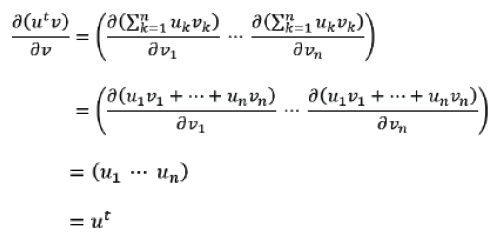and since 𝒰t𝒱 is a scalar, we are facing a particular case of the derivative of a scalar λ with respect to a vector, e.g., ∂𝒱λ=(∂𝒱1λ ..... ∂𝒱nλ) and it must also be ∂𝒱(𝒰t𝒱)=∂𝒱(𝒱t𝒰) . Moreover, it is easy to demonstrate that using the denominator layout, the derivative would have been ∂𝒱(𝒰t𝒱)=𝒰

If both 𝒰 and 𝒱 vectors are function of a third vector 𝒵, we get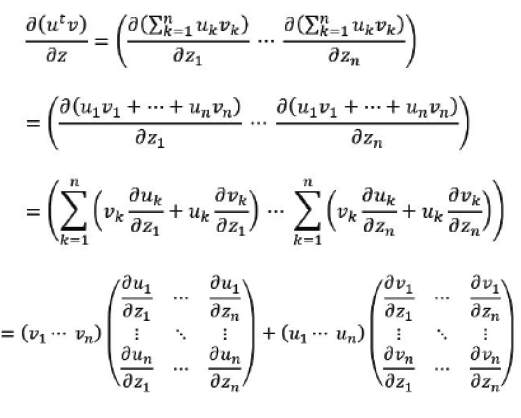which, in the case 𝒰=𝒱=𝒲 reduces toDealing with a linear transform 𝒰=a𝒱, if A is 𝓂×𝓂 we have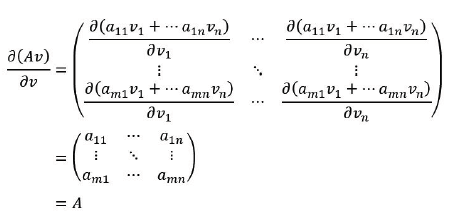and if 𝒱 is a function of a vector 𝒲𝒱 we get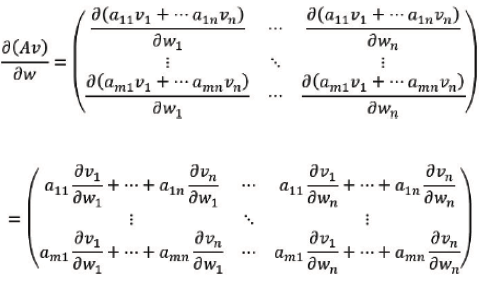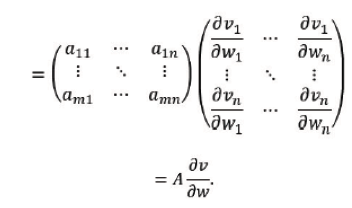From definition of bilinear form, we obtain, for 𝒰tA𝒱 the derivative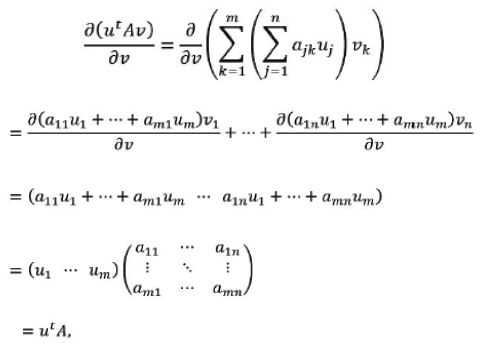while, for a quadratic form 𝒰tA𝒱(where A is 𝓃 × 𝓃), we get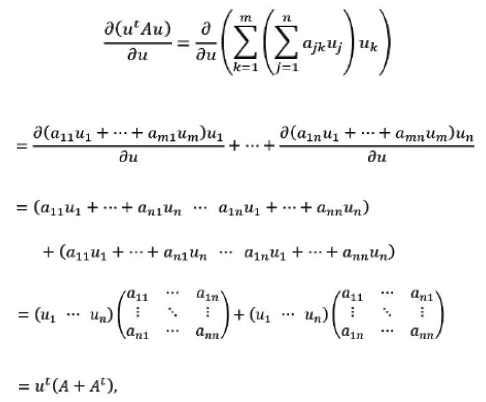Read More About Lupine Publishers Journal of Biostatistics & Biometrics Please Click on Below Link: https://lupine-publishers-biostatistics.blogspot.com/